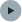Installation

Guide

Data

View

Time

Storage

HTTP

Route

Examples

# Time

The time driver provides the current time and allows scheduling application functions to run after specific times.

## Input

The time driver provides the current time as the number of milliseconds passed since January 1, 1970 00:00:00 UTC (the output of `Date.now()`).

``````Moon.use({
time: Moon.time.driver
});

Moon.run(({ time }) => {
console.log(time);
return {};
});``````

## Output

The time driver accepts a map from timeouts in milliseconds to application functions. It will run each application function after the specified time has elapsed.

``````Moon.use({
time: Moon.time.driver
});

Moon.run(() => ({
time: {
1000: () => ({}), // Runs after one second
2000: () => ({}), // Runs after two seconds
3000: () => ({}) // Runs after three seconds
}
}));``````

This can be useful to update a view, for example:

``````const p = Moon.view.m.p;

const main = ({ time }) => ({
view:
<p>
The time is {new Date(time).toLocaleTimeString()}.
</p>,
time: { 1000: main } // Recurse after one second.
});

Moon.use({
view: Moon.view.driver("#root"),
time: Moon.time.driver
});

Moon.run(main);``````